• +91 9971497814
• info@interviewmaterial.com

# Chapter 3 Trigonometric Functions Ex-3.2 Interview Questions Answers

### Related Subjects

Question 1 : Find the values of other five trigonometric functions if cos x = -1/2, x lies in third quadrant.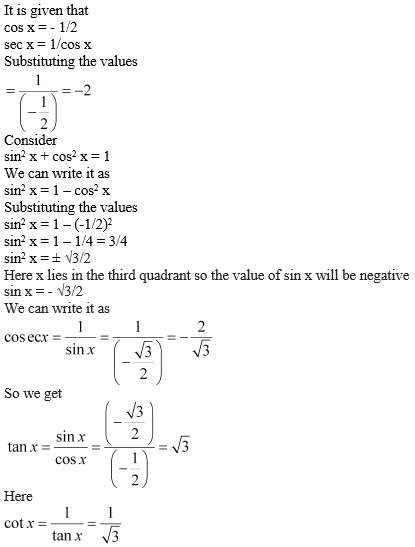Question 2 : Find the values of other five trigonometric functions if sin x = 3/5, x lies in second quadrant.

It is given that

sin x = 3/5

We can write it as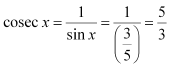We know that

sin2 x+ cos2 x = 1

We can write it as

cos2 x= 1 – sin2 x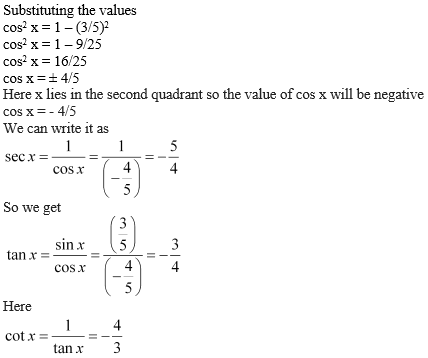Question 3 : Find the values of other five trigonometric functions if cot x = 3/4, x lies in third quadrant.

It is given that

cot x = 3/4

We can write it as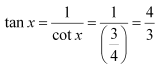We know that

1 + tan2 x= sec2 x

We can write it as

1 + (4/3)2 =sec2 x

Substituting thevalues

1 + 16/9 = sec2 x

cos2 x= 25/9

sec x = ± 5/3

Here x lies in thethird quadrant so the value of sec x will be negative

sec x = – 5/3

We can write it as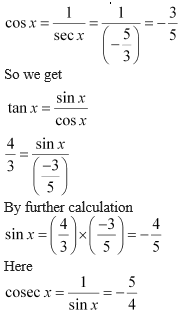Question 4 : Find the values of other five trigonometric functions if sec x = 13/5, x lies in fourth quadrant.

It is given that

sec x = 13/5

We can write it as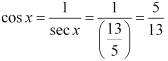We know that

sin2 x+ cos2 x = 1

We can write it as

sin2 x= 1 – cos2 x

Substituting thevalues

sin2 x= 1 – (5/13)2

sin2 x= 1 – 25/169 = 144/169

sin2 x= ± 12/13

Here x lies in thefourth quadrant so the value of sin x will be negative

sin x = – 12/13

We can write it as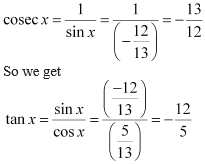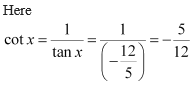Question 5 : Find the values of other five trigonometric functions if tan x = -5/12, x lies in second quadrant.

It is given that

tan x = – 5/12

We can write it as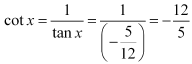We know that

1 + tan2 x= sec2 x

We can write it as

1 + (-5/12)2 =sec2 x

Substituting thevalues

1 + 25/144 = sec2 x

sec2 x= 169/144

sec x = ± 13/12

Here x lies in thesecond quadrant so the value of sec x will be negative

sec x = – 13/12

We can write it as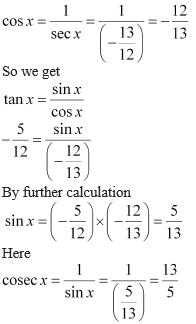Question 6 : Find the value of the trigonometric function sin 765°

We know that values ofsin x repeat after an interval of 2π or 360°

So we get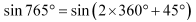By further calculation

= sin 45o

= 1/ √ 2

Question 7 : Find the value of the trigonometric function cosec (–1410°)

We know that values ofcosec x repeat after an interval of 2π or 360°

So we get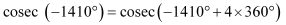By further calculation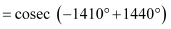= cosec 30o =2

Question 8 : Find the value of the trigonometric function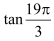We know that values oftan x repeat after an interval of π or 180°

So we get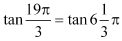By further calculation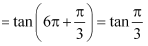We get

= tan 60o

= √3

Question 9 : Find the value of the trigonometric function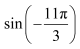We know that values ofsin x repeat after an interval of 2π or 360°

So we get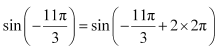By further calculation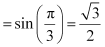Question 10 : Find the value of the trigonometric function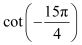We know that values oftan x repeat after an interval of π or 180°

So we get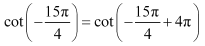By further calculation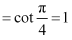Todays Deals### Chapter 3 Trigonometric Functions Ex-3.2 Contributorskrishan

Name:
Email:

# Latest News# 9000 interview questions in different categories